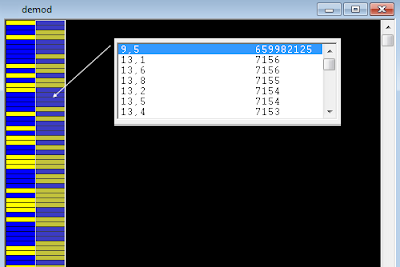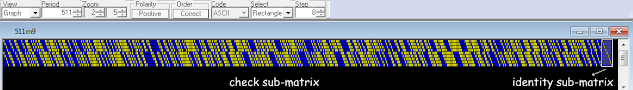## 12 March 2021

### Rus PSK4 1200Bd: solved

The questions related to the "nature" of the 1022-bit period of the QPSK 1200Bd signal (see the previous post) has been solved thanks to the help of my friend cryptomaster: I was waiting for traffic ("I tuned it waiting for traffic, but luckless until it went off") but actually it was already there!
He had the great intuition to reshape the bitstream into di-bit symbols, ie 2 bits per row given the 4ary modulation, then we gone on analyze the two columns separately and found the m-sequence x^9+x^5+1 (or the equivalent x^9+x^4+1) in the second column: therefore, each bit of data is followed by one bit of the m-sequence, ie the 1022-bit period consists of 511 bit of data interleaved with the 511 bit of the sequence generated by the LFSR x^9+x^5+1 (Figure 1).Fig. 1 - x^9+x^5+1 sequence in the 2nd column

The next step was to see if the same polynomial is to some extent involved in the bits of the first column. Based on the ninth degree of the polynomial x^9+x^5+1, a 9x511 parity check matrix was constructed, assuming that an H(511,502) code - based on that same polynomial - is used in the fomation of the bits of column #1. Notice that  a H(m,k) code is a Hamming code that encodes k bits of data into m bits (the codeword), adding m-k parity bits (CRC).
Our parity matrix consists of a 9x502 check sub-matrix and a 9x9 identity sub-matrix (Figure 2):Fig. 2 - Hamming parity check (511,502) matrix

As you know, the CRC formation is carried out by comparing column-by-column the k bits of each data row in turn with all the (m-k) rows of the check sub-matrix and counting the "vertical" correspondences of the "1"s of the data row and the r-row of the check sub-matix: if the count is odd then the CRC bit #r will be set to "1", otherwhise will be set to "0". That way, the (m-k) bits of the CRC is appended to the k bits of the data row just examined and the computation go ahead with the next data row.
That said, the bitstream of column #1 (the file demod-bit1.txt) has been reshaped to form a 511-column matrix of codewords. Manually checking the about two hundred 511-bit length codewords would have been a nightmare, so I wrote a short Octave script that would do the job for me and calculate the 9-bit CRC of each row along with a simulation of the final PSK4 modulation - the result in Figure 3.Fig. 3 - 9 bit CRC rows (left); PSK4 modulation of data +CRC (right)

By comparing the calculated CRC bits (Figure 3 on the left) with the bit stream of column n. 1 (Figure 4), it is clear that each row of the bitstream is nothing more than a "codeword" consisting of 502 bits for data plus 9 bits for Hamming CRC, ie the 511-bit period that we saw.Fig. 4 - the bistream of column #1

My friend cryptomaster tested also a 2016 recording and found it matches with the above conclusions (Figure 5). For what concerns the nature of the 502-bit strings of data they are probably telecontrols, further recordings are need. A possible (!) functional block diagram of the modulator is shown in Figure 6.